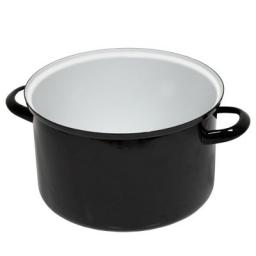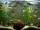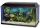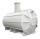# The pot

Diameter of the pot 38 cm. The height is 30 cm. How many liters of water can fit in the pot?

V =  34.02 l

### Step-by-step explanation:Did you find an error or inaccuracy? Feel free to write us. Thank you!Tips to related online calculators
Do you know the volume and unit volume, and want to convert volume units?

#### You need to know the following knowledge to solve this word math problem:

We encourage you to watch this tutorial video on this math problem:

## Related math problems and questions:

• Liters in cylinderDetermine the height at which level 24 liters of water in a cylindrical container having a bottom diameter 36 cm.
• Collect rain waterThe garden water tank has a cylindrical shape with a diameter of 80 cm and a height of 12 dm. How many liters of water will fit into the tank?
• ConvaHow many liters of water fit into the shape of a cylinder with a bottom diameter 20 cm and a height 45 cm?
• The potThe pot is a cylinder with a volume of V = 7l and an inner diameter of d = 20cm. Find its depth.
• The potThe pot is in 1/3 filled with water. Bottom of the pot has an area of ​​329 cm2. How many centimeters rises water level in the pot after add 1.2 liters of water?
• Water flow 2How many litres of water will flow in 7 minutes from a cylindrical pipe 1 cm in diameter, if the water flows at a speed of 30 km per hour
• SnailsHow many liters of water will fit in an aquarium with bottom dimensions of 30 cm and 25 cm and a height of 60 cm, if we pour water up to a height of 58 cm? How many most snails can we keep in an aquarium, if we know that snails need 600 cm ^ 3 of water fo
• PotCalculate the height of 3 liter pot with shape cylinder with a diameter of 10 cm.
• Tank and waterWe poured a cylindrical tank with 3.5 liters of water. If the tank base diameter is 3 dm, how height is the water level in?
• Water overflowA rectangular container that has a length of 30 cm, a width of 20 cm, and a height of 24 cm is filled with water to a depth of 15 cm. When an additional 6.5 liters of water are poured into the container, some water overflows. How many liters of water over
• PoolThe pool has the shape of a cylinder with a diameter of 4.6 m, and the presumed height is 130cm. The bucket fits 12l of water. How many buckets do you have to bring to fill this pool?
• Water cylinderZuzana poured 785 ml of water into a measuring cylinder with a base radius of 5 cm. The water in the cylinder reached a height of 2 cm from the upper edge of the cylinder. How tall is the cylinder? (π = 3.14)
• AquariumHow many liters of water can fit in an aquarium measuring 30, 15 and 20 cm?
• Cylinder:Cylinder: calculate the height of a 3 liter cylindrical pot with a diameter of 10 cm.
• Cuboid aquariumCuboid 25 times 30 cm. How long is third side if cuboid contains 30 liters of water?
• Diameter of a cylinderI need to calculate the cylinder volume with a height of 50 cm and a diameter of 30 cm.
• PebbleThe aquarium with internal dimensions of the bottom 40 cm × 35 cm and a height of 30 cm is filled with two-thirds of water. Calculate how many millimeters the water level in the aquarium rises by dipping a pebble-shaped sphere with a diameter of 18 cm.